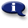# .

## Syllabus Information

Summer 2022
Dec 06,2023Use this page to maintain syllabus information, learning objectives, required materials, and technical requirements for the course.

Syllabus Information
MTH 065 - Elementary Algebra
Associated Term: Summer 2022
Learning Objectives: Upon successful completion of this course, the student will be able to:
1.Maintain, use, and expand skills and concepts learned in previous mathematics courses
a. Solve linear equations algebraically
b. Calculate slope of a line and find intercepts
c. Graph equations in two variables
d. Write equations in point-slope form and slope-intercept form
2. Solve linear systems of two equations in two unknowns
a. Solve algebraically and by graphing
b. Solve application problems involving linear systems of equations (Includes simple interest, motion, and mixture problems)
3. Evaluate and/or simplify expressions using the rules of (integer) exponents
4. Use scientific notation
5. Use the terminology of polynomials and add, subtract, multiply, and divide polynomials
a. Recognize and use the terminology of polynomials
b. Evaluate polynomials
c. Add, subtract, and multiply polynomials
d. Divide a polynomial by a monomial
6. Factor polynomials, including multivariable polynomials
a. Factor polynomials by removing a common monomial factor
b. Factor trinomials
c. Factor special products
Required Materials:
Technical Requirements: